## 引言

### Log-Gabor滤波器

Log-Gabor 滤波器的表达是从Gabor衍生而来的，相比于Gabor滤波器，它的传递函数是对数频率尺度下的高斯函数，始终没有直流分量，在图像处理时可以不受亮度的影响。

Log-Gabor的传递函数为：
G ( ω ) = e − log ⁡ ( ω / ω 0 ) 2 / ( 2 ( log ⁡ ( k / ω 0 ) 2 ) ) G(\omega)=e^{-\log(\omega/\omega_0)^2/(2(\log(k/\omega_0)^2))}
Log-Gabor函数并不能在空间域中得到表达式，滤波器的构造须在频域中。对图像来说，在空域的卷积等价于在频域的乘积，所以我们直接创建与图像大小相同的Log-Gabor滤波器，在频域中完成图像处理，之后再回到空域中进行统计。一个二维的Log-Gabor滤波器可以分解为径向滤波器和角度滤波器两部分，对应极坐标公式为：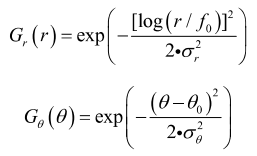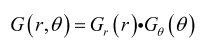### 构造滤波器

import cv2
import numpy as np
import matplotlib.pyplot as plt

n_scales=4
n_angles=6
H,W,_ = ir_img.shape

def lowpassfilter(H, W, cutoff, n):
if cutoff < 0 or cutoff > 0.5:
raise ValueError('the cutoff frequency needs to be between 0 and 0.5')

if not n == int(n) or n < 1.0:
raise ValueError('n must be an integer >= 1')

xrange = np.linspace(-0.5, 0.5, W)
yrange = np.linspace(-0.5, 0.5, H)

x, y = np.meshgrid(xrange, yrange)
radius = np.sqrt(x ** 2 + y ** 2)
return 1.0 / (1.0 + (radius / cutoff) ** (2 * n))

xrange = np.linspace(-0.5, 0.5, W)
yrange = np.linspace(-0.5, 0.5, H)
x, y = np.meshgrid(xrange, yrange)
radius = np.sqrt(x ** 2 + y ** 2)
theta = np.arctan2(-y, x)

# numpy.fft模块中的fftshift函数可以将FFT输出中的直流分量移动到频谱的中央。ifftshift函数则是其逆操作
theta = np.fft.ifftshift(theta)

sintheta = np.sin(theta)
costheta = np.cos(theta)

lp_filter = lowpassfilter(H, W, 0.45, 15)
# lp_filter = np.fft.ifftshift(lp_filter)
log_gabor_filters = np.zeros((n_scales, H, W))

#  不同尺度
for sc in range(n_scales):
wavelength = min_wavelength * multiplier ** sc
log_gabor_filters[sc] = np.exp(
(-(np.log(radius * wavelength + 1e-5)) ** 2) / (2 * np.log(sigma_onf + 1e-5) ** 2)) * lp_filter

#  不同方向
for o in range(n_angles):
angle = o * np.pi / n_angles
ds = sintheta * np.cos(angle) - costheta * np.sin(angle)
dc = costheta * np.cos(angle) + sintheta * np.sin(angle)
dtheta = abs(np.arctan2(ds, dc))
dtheta = np.minimum(dtheta * n_angles * 0.5, np.pi)
spreads[o] = (np.cos(dtheta) + 1) / 2
#  构造集合的filter
filter_bank = np.zeros((n_scales * n_angles, H, W))
for sc in range(n_scales):
for o in range(n_angles):
filter_bank[sc * n_angles + o] = log_gabor_filters[sc] * spreads[o]

#  可视化
for sc in range(n_scales):
plt.figure(sc,figsize=(30,120))
first = filter_bank[sc * n_angles] - np.min(filter_bank[sc * n_angles])
first /= np.max(first)
first *= 255
shuiping = first
for o in range(n_angles-1):
out = filter_bank[sc * n_angles + o+1] - np.min(filter_bank[sc * n_angles + o+1])
out /= np.max(out)
out *= 255
shuiping = np.hstack((shuiping,out))
plt.imshow(shuiping,cmap="gray")


### 滤波器可视化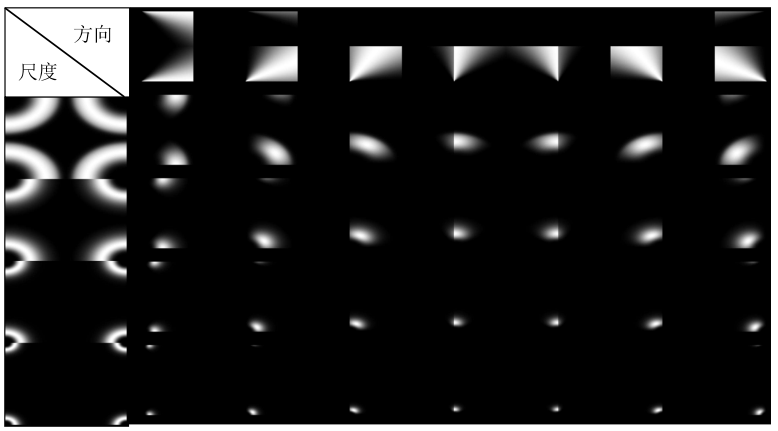### 进行图像测试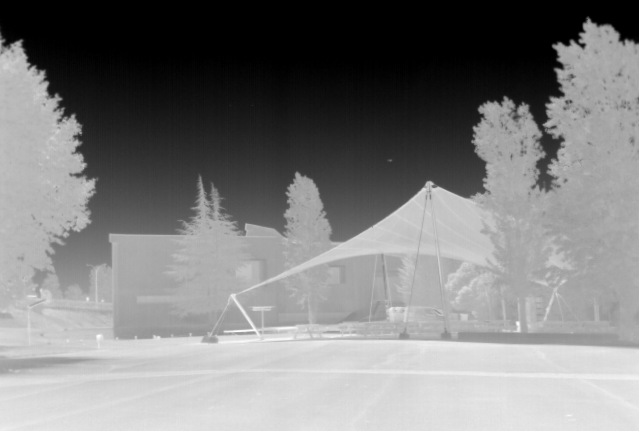vi_gray = cv2.cvtColor(img, cv2.COLOR_BGR2GRAY)
# 图像的傅里叶变换
image_fft = cv2.dft(np.float32(vi_gray), flags=cv2.DFT_COMPLEX_OUTPUT)
eo = np.zeros((filter_bank.shape, filter_bank.shape, filter_bank.shape, 2))
for i, filter in enumerate(filter_bank):
eo[i] = cv2.idft(np.multiply(np.expand_dims(filter, -1), image_fft))

for sc in range(n_scales):
plt.figure(sc,figsize=(30,120))
first = eo_magnitude[n_scales]
first /= np.max(first)
first *= 255
shuiping = first
for o in range(n_angles-1):
out = eo_magnitude[sc * n_angles + o+1] - np.min(eo_magnitude[sc * n_angles + o+1])
out /= np.max(out)
out *= 255
shuiping = np.hstack((shuiping,out))
plt.imshow(shuiping,cmap="gray")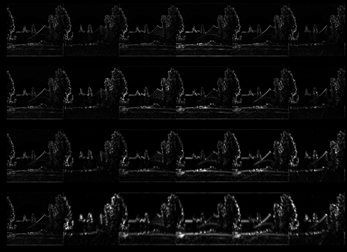### Apache CXF - 快速指南_allway2的博客-程序员秘密

Apache CXF - 简介在当今的环境中，您可以使用多个选项来创建 Web 服务应用程序。您可以使用多种标准和广泛接受的协议中的一种或多种进行通信。例如SOAP、XML/HTTP、RESTful HTTP和CORBA（通用对象请求代理架构，在过去非常流行，但现在不常用。您还可以选择不同的传输方式，例如 HTTP、JMS、JBI和前端 API，例如JAX-RS和JAX-WS。有这么多的 Web 服务开发选项，需要一个开源服务框架来将上述所有选项粘合在一起，这就是Apache CXF所做的。在本

### &nbsp是jsp里的空格_balei2634的博客-程序员秘密

&amp;nbsp 在jsp里是空格的意思，jsp里如果用连续空格会被认为是一个，用个&amp;nbsp可以表达多少空格。转载于:https://www.cnblogs.com/youwuyi/p/10233278.html...

### CocoaPods安装和使用教程_太阳火神的美丽人生的博客-程序员秘密

CocoaPods安装和使用教程 Code4App 原创文章。转载请注明出处：http://code4app.com/article/cocoapods-install-usage目录CocoaPods是什么？如何下载和安装CocoaPods？如何使用CocoaPods？ 场景1：利用CocoaPods，在项目中导入AFNetworking类库场景2：如何正确编译运行一个包含C

### SAP-abap-OLE核心代码_abap ole_Qunending的博客-程序员秘密

OLE定义DATA: stexcl TYPE ole2_object, stwkli TYPE ole2_object, stappl TYPE ole2_object, stwkbk TYPE ole2_object, stacts TYPE ole2_object, stshet TYPE ole2_object, stnush TYPE ole2_object, stnunm TYPE ole2_object,